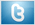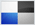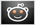# Analyzing Progressions To Find The Proper Scale II

For the first example, lets assume that you have determined the song is in the key of E, and also uses the F, G, A, B and C notes. Have a look at the diagram below.This one is almost analyzed for us already and I'll bet you can see what it looks like. The first and easiest way to analyze this is to simply look at the pattern. You may see what modal position it looks like.

Is there any way the scale for a song using these notes could be E Ionian ? It should be clear why this could not be E Ionian but I will explain. If you already know the intervals for each scale (and you should), you would know that E Ionian would start with the E note, and then be followed by F# and G#. So it could not be the E major scale as our scale uses F and G. Simply imagine playing the Ionian position starting on the E and you'll see that it would not work.

So what else can we determine here using the same method ? We can see that this scale starts off with the root, minor 2nd, and the minor 3rd. If you know your modes and their intervals, you will see that there are only two modes that this example could be. The minor 2nd here is the key to this, as this note is only present in two of our modes. These modes would be Phrygian and Locrian. Every other mode that you have learned used the major 2nd, except for Phrygian and Locrian.

So which of these two is it ? The Locrian position would be root (E), minor 2 (F), minor 3 (G), perf 4 (A), dim 5 (A#), minor 6 (C). That clearly couldn't be it, because this scale does not use the A# note, it uses the B. Phrygian would use all of the same notes as locrian except for that A# where phrygian would use the B.

So there you have it, this would be E Phrygian based on the notes we see being used. Since we have a lot of the notes being used, you may have recognized the phrygian position here already. In the next one, we will work with fewer notes to make it a little less recognizable. If you still don't understand how this is done, don't worry, more will be explained in the next lesson.

Now lets move on to the next lesson and try a little more advanced analyzing, which will teach us some new ways to determine the scale.

••••••